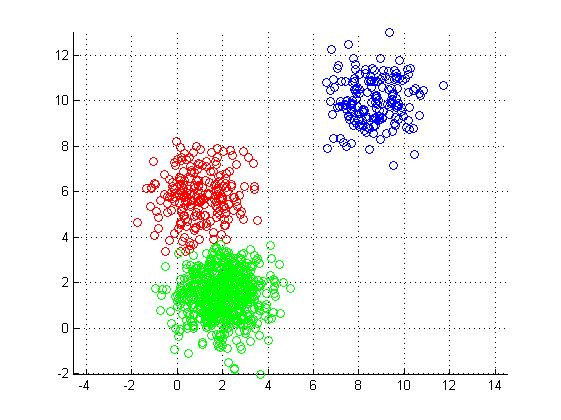# K-Means Clustering

There are times in research when you neither want to predict nor classify examples. Rather, you want to take a dataset and segment the examples within the dataset so that examples with similar characteristics are gathered together.

When your goal is to great several homogeneous groups within a sample it involves clustering. One type of clustering used in machine learning is k-means clustering. In this post, we will learn the following about k-means clustering.

• The purpose of k-means
• Pros and cons of k-means

The Purpose of K-means

K-means has a number of applications. In the business setting, k-means has beenused to segment customers. Businesses use this information to adjusts various aspects of their strategy for reaching their customers.

Another purpose for k-means is in simplifying large datasets so that you have several smaller datasets with similar characteristics. This subsetting could be useful in finding distinct patterns

K-means is a form of unsupervised classification. This means that the results label examples that the researcher must give meaning too. When R gives the results of an analysis it just labels the clusters as 1,2,3 etc. It is the researcher’s job to look at the clusters and give a qualitative meaning to them.

Pros and Cons of K-Means

The pros of k-means is that it is simple, highly flexible, and efficient. The simplicity of k-means makes it easy to explain the results in contrast to artificial neural networks or support vector machines. The flexibility of k-means allows for easy adjust if there are problems. Lastly, the efficiency of k-means implies that the algorithm is good at segmenting a dataset.

Some drawbacks to k-means are that it does not allow to develop the most optimal set of clusters and that the number of clusters to make must be decided before the analysis. When doing the analysis, the k-means algorithm will randomly select several different places from which to develop clusters. This can be good or bad depending on where the algorithm chooses to begin at. From there, the center of the clusters is recalculated until an adequate “center” is found for the number of clusters requested.

How many clusters to include is left at the discretion of the researcher. This involves a combination of common sense, domain knowledge, and statistical tools. Too many clusters tell you nothing because the groups becoming very small and there are too many of them.

There are statistical tools that measure within-group homogeneity and with group heterogeneity. In addition, there is a technique called a dendrogram. The results of a dendrogram analysis provide a recommendation of how many clusters to use. However, calculating a dendrogram for a large dataset could potential crash a computer due to the computational load and the limits of RAM.

Conclusion.

K-means is an analytical tool that helps to separate apples from oranges to give you one example. If you are in need of labeling examples based on the features in the dataset this method can be useful.